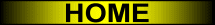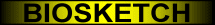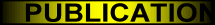Force Histograms Computed in O(NlogN)
ABSTRACT
 The relative position between two objects in a 2D raster image is often represented quantitatively by a force histogram. In the general case, force histograms are computed in O(KN√N) time: N is the number of pixels in the image and K is the number of directions in which forces are considered. When the objects are defined as fuzzy sets, this complexity also depends quadratically on the number M of possible membership degrees. In the present paper, an algorithm that runs in O(NlogN) is introduced. Computation times are basically independent of K and M. All objects (convex, concave, crisp, fuzzy) are handled in an equally fast manner. Experiments validate the theoretical analysis.